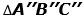# U1B Test

## Use the information to answer questions 12 and 13. triangleABC has vertices A(-1, 0), B(4, 0), C(2, 6)

What are the the coordinates for each vertex?

## Use the information to answer questions 12 and 13. triangleABC has vertices A(-1, 0), B(4, 0), C(2, 6)

Write the function rule (xy) → ________ to create. What are the coordinates for each vertex?